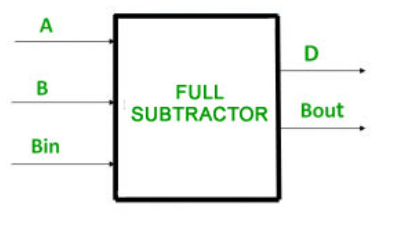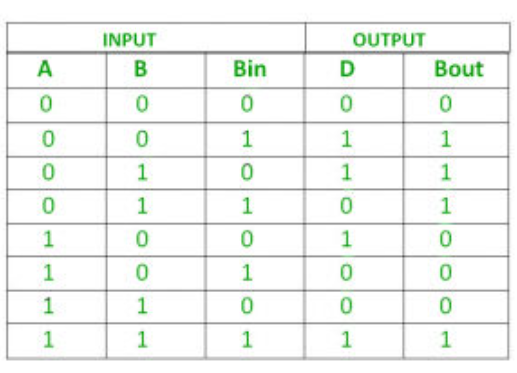Related Articles

# C++ program to implement full subtractor

• Difficulty Level : Expert
• Last Updated : 17 Aug, 2021

Prerequisite : Full Subtractor
Given three inputs of Full Subtractor A, B, Bin. The task is to implement the Full Subtractor circuit and Print output states difference (D) and Bout of three inputs.

Introduction :
The full subtractor is used to subtract three 1-bit numbers  which are minuend, subtrahend, and borrow, respectively. The full subtractor has three input states and two output states. The two outputs are difference and borrow.Here we have three inputs A, B, Bin and two outputs D, Bout. And the truth table for full subtractor isLogical Expression :

```Difference = (A XOR B) XOR Bin
Borrow Out = Ā Bin + Ā B + B Bin```

Examples –

Input         :    0 0 1
Output      :     Difference=1, B-Out=1
Explanation :   According to logical expression Difference= (A XOR B) XOR Bin  i.e (0 XOR 0) XOR 1  =1 ,
B-Out=Ā Bin + Ā B + B Bin  i.e.  1AND 1 + 1 AND 0 + 0 AND 1 = 1.

Input         :    1 0 1
Output      :     Difference=0, B-Out=0

Approach :

• We will take three inputs A, B, Bin.
• By applying (A XOR B) XOR Bin gives the Value of Difference.
• By applying Ā Bin + Ā B + B Bin gives the value of B-Out.

## C++

 `//c++ code for above approach``#include ``using` `namespace` `std;``//function to print difference and borrow out``void` `Full_Subtractor(``int` `A,``int` `B,``int` `Bin){``  ` `   ``// Calculating value of Difference``    ``int` `Difference = (A ^ B) ^ Bin;``      ` `    ``//calculating NOT  value of a``    ``int` `A1 = not(A);``      ` `    ``//Calculating value of B-Out``    ``int` `B_Out = A1 & Bin | A1 & B | B & Bin;``  ` `    ``//printing the values``    ``cout<<``"Difference = "``<
Output
```Difference = 0
B-Out = 0
```

Attention reader! Don’t stop learning now. Get hold of all the important CS Theory concepts for SDE interviews with the CS Theory Course at a student-friendly price and become industry ready.

My Personal Notes arrow_drop_up